# IF Formula – If Then Statements – Excel & Google Sheets

This tutorial demonstrates how to use the IF Function in Excel and Google Sheets to create If Then Statements.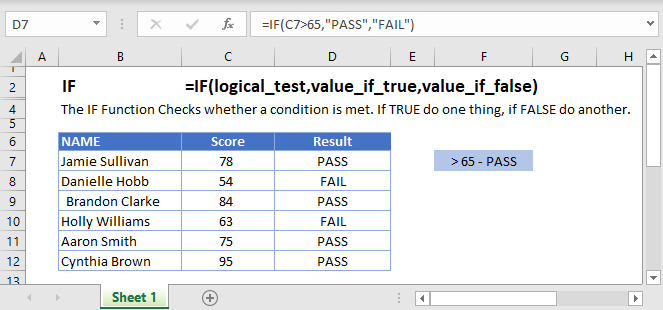## IF Function Overview

The IF Function Checks whether a condition is met. If TRUE do one thing, if FALSE do another.

## How to Use the IF Function

Here’s a very basic example so you can see what I mean. Try typing the following into Excel:

``=IF( 2 + 2 = 4,"It’s true", "It’s false!")``

Since 2 + 2 does in fact equal 4, Excel will return “It’s true!”. If we used this:

``=IF( 2 + 2 = 5,"It’s true", "It’s false!")``

Now Excel will return “It’s false!”, because 2 + 2 does not equal 5.

Here’s how you might use the IF statement in a spreadsheet.

``=IF(C4-D4>0,C4-D4,0)``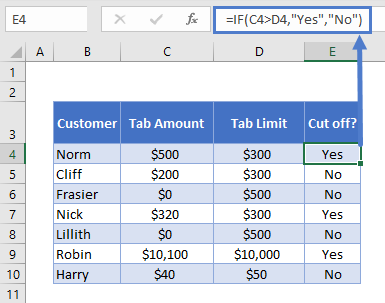You run a sports bar and you set individual tab limits for different customers. You’ve set up this spreadsheet to check if each customer is over their limit, in which case you’ll cut them off until they pay their tab.

You check if C4-D4 (their current tab amount minus their limit), is greater than 0. This is your logical test. If this is true, IF returns “Yes” – you should cut them off. If this is false, IF returns “No” – you let them keep drinking.

## What can IF Return?

Above we returned a text string, “Yes” or “No”. But you can also return numbers, or even other formulas.

Let’s say some of your customers are running up big tabs. To discourage this, you’re going to start charging interest on customers who go over their limit.

You can use IF for that:

``=IF(C4>D4,C4*0.03,0)``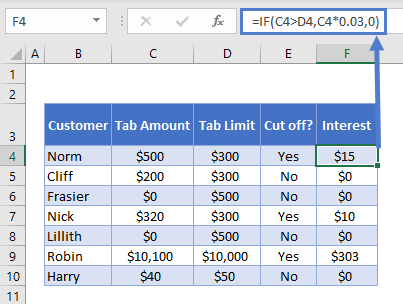If the tab is higher than the limit, return the tab multiplied by 0.03, which returns 3% of the tab. Otherwise, return 0: they aren’t over their tab, so you won’t charge interest.

## Using IF with AND

You can combine IF with Excel’s AND Function to test more than one condition. Excel will only return TRUE if ALL of the tests are true.

So, you implemented your interest rate. But some of your regulars are complaining. They’ve always paid their tabs in the past, why are you cracking down on them now? You come up with a solution: you won’t charge interest to certain trusted customers.

You make a new column to your spreadsheet to identify trusted customers, and update your IF statement with an AND function:

``=IF(AND(C4>D4, F4="No"),C4*0.03,0)``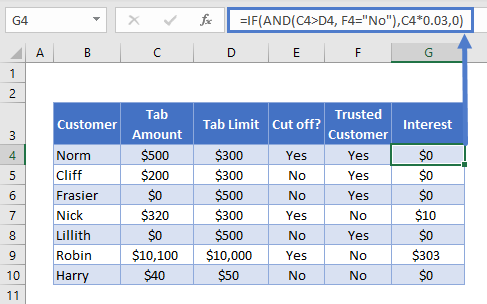Let’s look at the AND part separately:

``AND(C4>D4, F4="No")``

Note the two conditions:

• C4>D4: checking if they’re over their tab limit, as before
• F4=“No“: this is the new bit, checking if they are not a trusted customer

So now we only return the interest rate if the customer is over their tab, AND we have “No” in the trusted customer column. Your regulars are happy again.

## Using IF with OR

The OR Function allows you to test more than one condition, returning TRUE if any conditions are met.

Maybe customers being over their tab is not the only reason you’d cut them off. Maybe you give some people a temporary ban for other reasons, gambling on the premises perhaps.

So you add a new column to identify banned customers, and update your “Cut off?” column with an OR test:

``=IF(OR(C4>D4,E4="Yes"),"Yes","No")``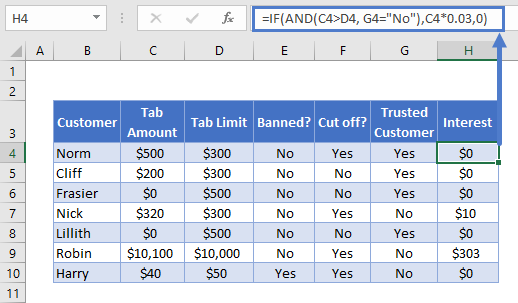Looking just at the OR part:

``OR(C4>D4,E4="Yes")``

There are two conditions:

• C4>D4: checking if they’re over their tab limit
• F4=“Yes“: the new part, checking if they are currently banned

This will evaluate to true if they are over their tab, or if there is a “Yes” in column E. As you can see, Harry is cut off now, even though he’s not over his tab limit.

## Using IF with XOR

The XOR Function returns TRUE if only one condition is met. If more than one condition is met (or not conditions are met). It returns FALSE.

An example might make this clearer. Imagine you want to start giving monthly bonuses to your staff :

• If they sell over \$800 in food, or over \$800 in drinks, you’ll give them a half bonus
• If they sell over \$800 in both, you’ll give them a full bonus
• If they sell under \$800 in both, they don’t get any bonus.

You already know how to work out if they get the full bonus. You’d just use IF with AND, as described earlier.

``=IF(AND(C4>800,D4>800),"Yes","No")``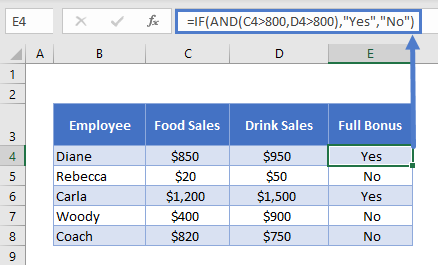But how would you work out who gets the half bonus? That’s where XOR comes in:

``=IF(XOR(C4>=800,D4>=800),"Yes","No")``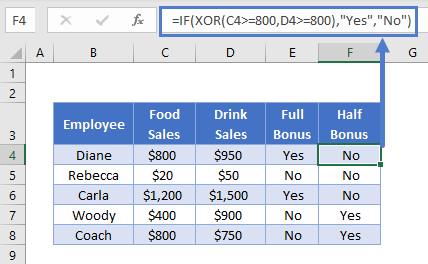As you can see, Woody’s drink sales were over \$800, but not food sales. So he gets the half bonus. The reverse is true for Coach. Diane and Carla sold more than \$800 for both, so they don’t get a half bonus (both arguments are TRUE), and Rebecca made under the threshold for both (both arguments FALSE), so the formula again returns “No”.

## Using IF with NOT

The NOT Function reverses the outcome of a logical test. In other words, it checks whether a condition has not been met.

You can use it with IF like this:

``=IF(AND(C3>=1985,NOT(D3="Steven Spielberg")),"Watch", "Don’t Watch")``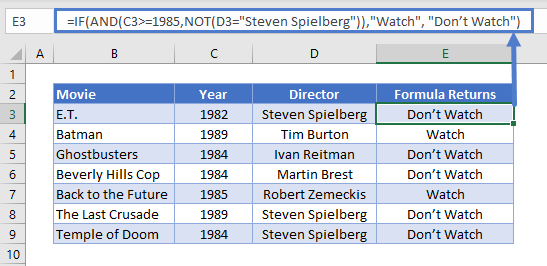Here we have a table with data on some 1980s movies. We want to identify movies released on or after 1985, that were not directed by Steven Spielberg.

Because NOT is nested within an AND Function, Excel will evaluate that first. It will then use the result as part of the AND.

## Nested IF Statements

You can also return an IF statement within your IF statement. This enables you to make more complex calculations.

Let’s go back to our customers table. Imagine you want to classify customers based on their debt level to you:

• \$0: None
• Up to \$500: Low
• \$500 to \$1000: Medium
• Over \$1000: High

You can do this by “nesting” IF statements:

``=IF(C4=0,"None",IF(C4<=500,"Low",IF(C4<=1000,"Medium",IF(C4>1000,"High"))))``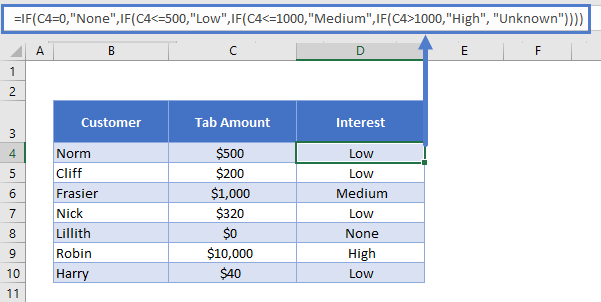It’s easier to understand if you put the IF statements on separate lines (ALT + ENTER on Windows, CTRL + COMMAND + ENTER on Macs):

``````=
IF(C4=0,"None",
IF(C4<=500,"Low",
IF(C4<=1000,"Medium",
IF(C4>1000,"High", "Unknown"))))``````

IF C4 is 0, we return “None”. Otherwise, we move to the next IF statement. IF C4 is equal to or less than 500, we return “Low”. Otherwise, we move on to the next IF statement… and so on.

## Simplifying Complex IF Statements with Helper Columns

If you have multiple nested IF statements, and you’re throwing in logic functions too, your formulas can become very hard to read, test, and update.

This is especially important to keep in mind if other people will be using the spreadsheet. What makes sense in your head, might not be so obvious to others.

Helper columns are a great way around this issue.

You’re an analyst in the finance department of a large corporation. You’ve been asked to create a spreadsheet that checks whether each employee is eligible for the company pension.

Here’s the criteria: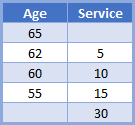So if you’re under the age of 55, you need to have 30 years’ service under your belt to be eligible. If you’re aged 55 to 59, you need 15 years’ service. And so on, up to age 65, where you’re eligible no matter how long you’ve worked there.

You could use a single, complex IF statement to solve this problem:

``=IF(OR(F4>=65,AND(F4>=62,G4>=5),AND(F4>=60,G4>=10),AND(F4>=55,G4>=15),G4>30),"Eligible", "Not Eligible")``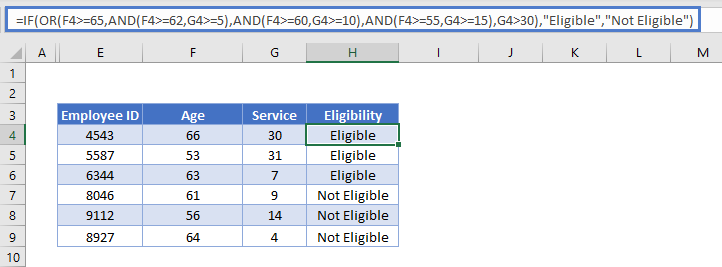A better approach might be to use helper columns. We have five logical tests here, corresponding to each row in the criteria table. This is easier to see if we add line breaks to the formula, as we discussed earlier:

``````=IF(
OR(
F4>=65,
AND(F4>=62,G4>=5),
AND(F4>=60,G4>=10),
AND(F4>=55,G4>=15),
G4>30
),"Eligible","Not Eligible")``````

So, we can split these five tests into separate columns, and then simply check whether any one of them is true: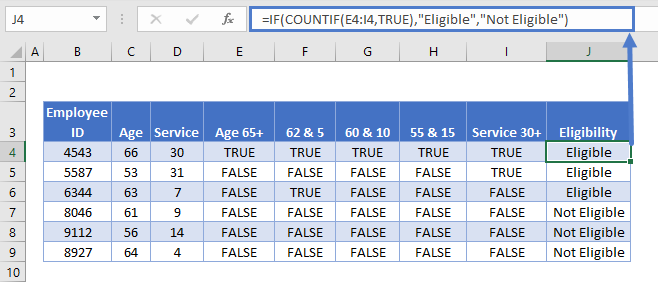Each column in the table from E to I holds each of our criteria separately. Then in J4 we have the following formula:

``=IF(COUNTIF(E4:I4,TRUE),"Eligible","Not Eligible")``

Here we have an IF statement, and the logical test uses COUNTIF to count the number of cells within E4:I4 that contain TRUE.

If COUNTIF doesn’t find a TRUE value, it will return 0, which IF interprets as FALSE, so the IF returns “Not Eligible”.

If COUNTIF does find any TRUE values, it will return the number of them. IF interprets any number other than 0 as TRUE, so it returns “Eligible”.

Splitting out the logical tests in this way makes the formula easier to read, and if something’s going wrong with it, it’s much easier to spot where the mistake is.

## Using Grouping to Hide Helper Columns

Helper columns make the formula easier to manage, but once you’ve got them in place and you know they are working correctly, they often just take up space on your spreadsheet without adding any useful information.

You could hide the columns, but this can lead to problems because hidden columns are hard to detect, unless you look closely at the column headers.

A better option is grouping.

Select the columns you want to group, in our case E:I. Then press ALT + SHIFT + RIGHT ARROW on Windows, or COMMAND + SHIFT + K on Mac. You can also go to the “Data” tab on the ribbon and select “Group” from the “Outline” section.

You’ll see the group displayed above the column headers, like this: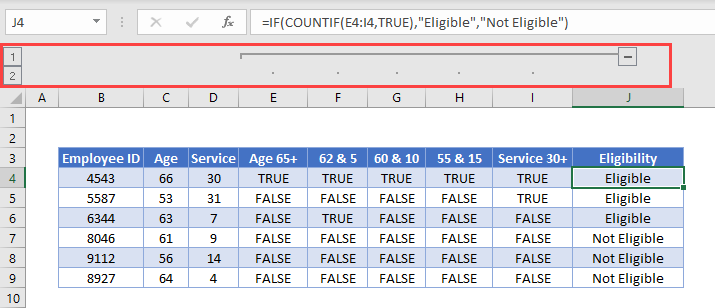Then simply press the “-“ button to hide the columns: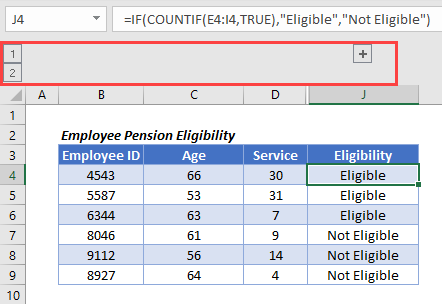## The IFS Function

Nested IF statements are very useful when you need to perform more complex logical comparisons, and you need to do it in one cell. However, they can get complicated as they get longer, and they can be hard to read and update on your screen.

From Excel 2019 and Excel 365, Microsoft introduced another function, the IFS Function, to help make this a bit easier to manage. The nested IF example above could be achieved with IFS like this:

``````=IFS(
C4=0,"None",
C4<=500,"Low",
C4<=1000,"Medium",
C4>1000,"High",
TRUE, "Unknown",
)``````

## Using IF with Conditional Formatting

Excel’s Conditional Formatting feature enables you to format a cell in different ways depending on its contents. Since the IF returns different values based on our logical test, we might want to use Conditional Formatting with the IF Function to make these different values easier to see.

So let’s go back to our staff bonus table from earlier.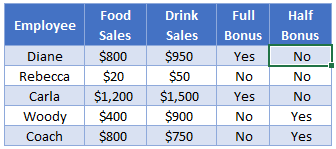We’re returning “Yes” or “No” depending on what bonus we want to give. This tells us what we need to know, but the information doesn’t jump out at us. Let’s try to fix that.

Here’s how you’d do it:

• Select the cell range containing your IF statements. In our case that’s E4:F8.
• Click “Conditional Formatting” on the “Styles” section of the “Home” tab on the ribbon.
• Click “Highlight Cells Rules” and then “Equal to”.
• Type “Yes” (or whatever return value you need) into the first box, and then choose the formatting you want from the second box. (I’ll choose green for this).
• Repeat for all your return values (I’ll also set “No” values to red)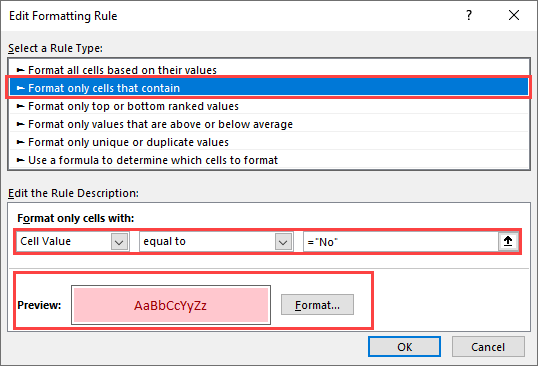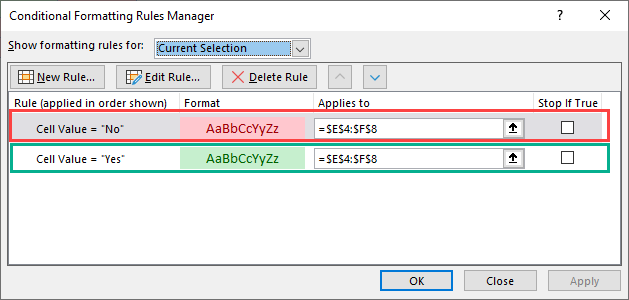Here’s the result: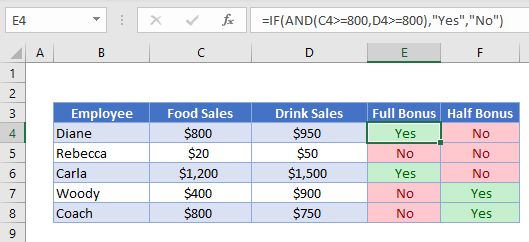## Using IF in Array Formulas

An array is a range of values, and in Excel arrays are represented as comma separated values enclosed in braces, such as:

``{1,2,3,4,5}``

The beauty of arrays, is that they enable you to perform a calculation on each value in the range, and then return the result. For example, the SUMPRODUCT Function takes two arrays, multiplies them together, and sums the results.

So this formula:

``=SUMPRODUCT({1,2,3},{4,5,6})``

…returns 32. Why? Let’s work it through:

``````1 * 4 = 4
2 * 5 = 10
3 * 6 = 18

4 + 10 + 18 = 32``````

We can bring an IF statement into this picture, so that each of these multiplications only happens if a logical test returns true.

For example, take this data: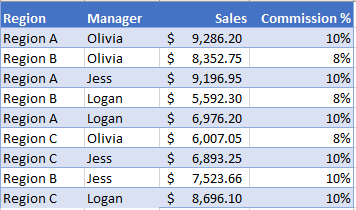If you wanted to calculate the total commission for each sales manager, you’d use the following:

``=SUMPRODUCT(IF(\$C\$2:\$C\$10=\$G2,\$D\$2:\$D\$10*\$E\$2:\$E\$10))``

Note: In Excel 2019 and earlier, you have to press CTRL + SHIFT + ENTER to turn this into an array formula.

We’d end up with something like this: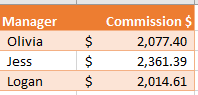Breaking this down, the “Manager” column is column C, and in this example, Olivia’s name is in G2.

So the logical test is:

``\$C\$2:\$C\$10=\$G2``

In English, if the name in column C is equal to what’s in G2 (“Olivia”), DO multiply the values in columns D and E for that row. Otherwise, don’t multiply them. Then, sum all the results.

The IF Function works exactly the same in Google Sheets as in Excel: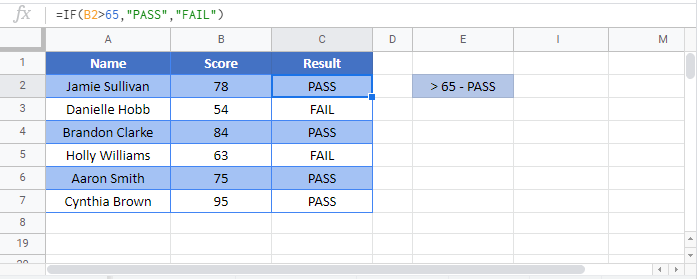## VBA IF Statements

You can also use If Statements in VBA. Click the link to learn more, but here is a simple example:

``````Sub Test_IF ()

If Range("a1").Value < 0 then
Range("b1").Value = "Negative"
End If

End If``````

This code will test if a cell value is negative. If so, it will write „negative“ in the next cell.